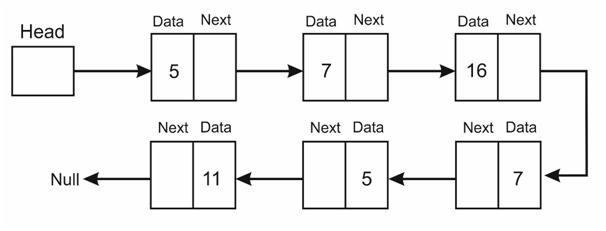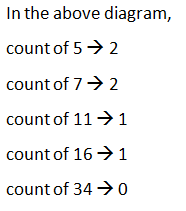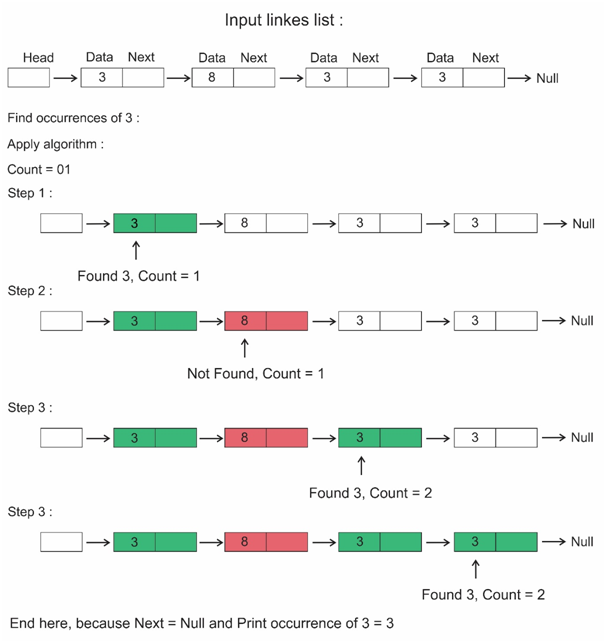# Total number of occurrences of a given item in the linked list

## Find the number of occurrences of a number in the given linked list

Occurrences : number of times x is coming.

### Example## Algorithm

Time Complexity : O(n)
Space Complexity : O(1)

Step 1 : Create a function which takes a linked list, a number as arguments and give the count of the number in the given linked list.
Step 2 : Initialize count equal to 0.
Step 3 : Traverse in Linked List, compare with the given number, if found a number equal to it, update count.
a)    If the element data equal to the required number increment count.
b)    After reaching the end of the Linked List return count.
Step 4 : call the function on given linked list and number you want to know occurrences. It prints the number of occurrences.

### Algorithm Working Example## C++ Program

```#include <bits/stdc++.h>
using namespace std;

struct LL{
int data;
LL *next;
};

{
struct LL* curr=new LL;

curr->data=dataToBeInserted;
curr->next=NULL;

*head=curr; //if this is first node make this as head of list

else
{
curr->next=*head; //else make the current (new) node's next point to head and make this new node a the head
}

//O(1) constant time
}

{
int count=0;
while(temp!=NULL)
{
if(temp->data == X)
count++;

temp=temp->next;
}

return count;

//O(number of nodes)
}

{
while(temp!=NULL)
{
if(temp->next!=NULL)
cout<<temp->data<<" ->";
else
cout<<temp->data;

temp=temp->next; //move to next node
}
//O(number of nodes)
cout<<endl;
}

int main()
{

struct LL *head = NULL; //initial list has no elements